# Filters Notes | Study Analog Electronics - Electrical Engineering (EE)

## Electrical Engineering (EE): Filters Notes | Study Analog Electronics - Electrical Engineering (EE)

The document Filters Notes | Study Analog Electronics - Electrical Engineering (EE) is a part of the Electrical Engineering (EE) Course Analog Electronics.
All you need of Electrical Engineering (EE) at this link: Electrical Engineering (EE)

FILTERS

The output of a rectifier contains dc component as well as ac component. Filters are used to minimize the undesirable ac i.e., ripple leaving only the dc component to appear at the output.

Some important filters are:

1. Inductor filter
2. Capacitor filter
3. LC or L section filter
4. CLC or Π-type filter

CAPACITOR FILTER

This is the most simple form of the filter circuit and in this arrangement a high value capacitor C is placed directly across the output terminals, as shown in figure. During the conduction period, it gets charged and stores energy in it. during non-conduction period. Through this process, the time duration during which Ft is to be noted here that the capacitor C gets charged to the peak because there is no resistance (except the negligible forward resistance of diode) in the charging path. But the discharging time is quite large (roughly 100 times more than the charging time depending upon the value of RL) because it discharges through load resistance RL.

The function of the capacitor filter may be viewed in terms of impedances. The large value capacitor C offers a low impedance shunt path to the ac components or ripples but offers high impedance to the dc component. Thus ripples get bypassed through capacitor C and only dc component flows through the load resistance RL.

Capacitor filter is very popular because of its low cost, small size, light weight and good characteristics.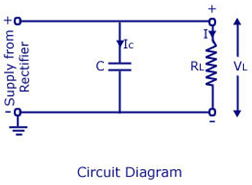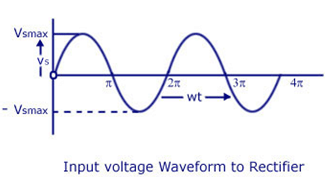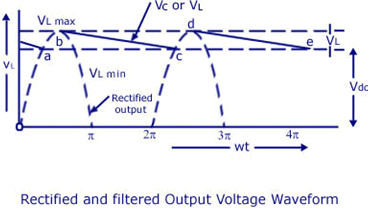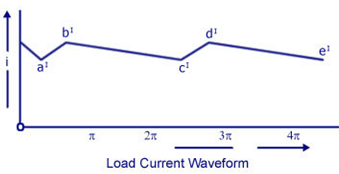CAPACITOR FILTER WITH HALF-WAVE RECTIFIER: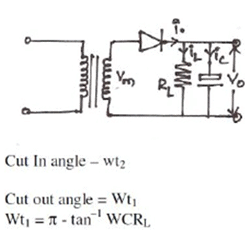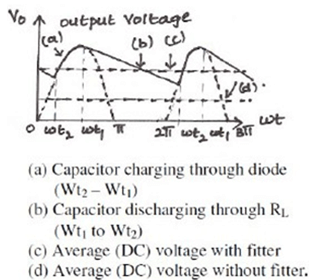CAPACITOR FILTER WITH FULL-WAVE RECTIFIER: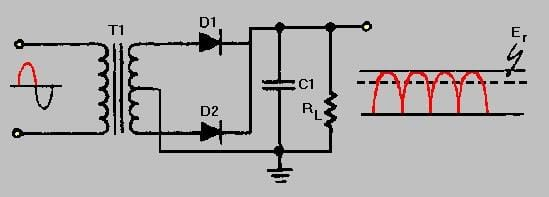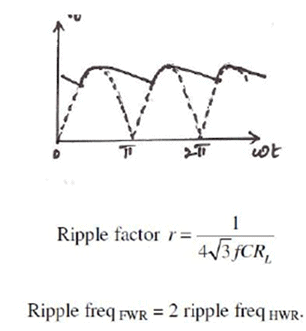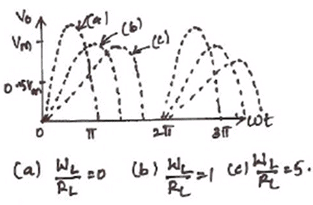### The worth noting points about shunt capacitor filter are:

1.For a fixed-value filter capacitance, larger the load resistance RL larger will be the discharge time constant CRL and therefore, lower the ripples more the output voltage. On the other hand lower the load resistance (or more the load current), lower will be the output voltage.

2.Similarly smaller the filter capacitor, the less charge it can hold and more it will discharge. Thus the peak-to-peak value of the ripple will increase, and the average dc level will decrease. Larger the filter capacitor, the more charge it can hold and the less it will discharge. Hence the peak-to-peak value of the ripple will be less, and the average dc level will increase. But, the maximum value of the capacitance that can be employed is limited by another factor. The larger the capacitance value, the greater is the current required to charge the capacitor to a given voltage. The maximum current that can be handled by a diode is limited by the figure quoted by the manufacturer. Thus the maximum value of the capacitance, that can be used in the shunt filter capacitor is limited.

### Series Inductor Filter:In this arrange­ment, a high value inductor or choke L is connected in series with the rectifier element and the load, as illustrated in figure. The filtering action of an inductor filter de­pends on its property of opposing any change in the current flowing through it. When the output current of the rectifier increases above a certain value, energy is stored in it in the form of magnetic field and this energy is given up when the output current falls below the average value. Thus by placing a choke coil in series with the rectifier output and load, any sudden change in current that might have occurred in the circuit without an inductor is smoothed out by the presence of the inductor L.

The function of the inductor filter may be viewed in terms of impedances. The choke offers high impedance to the ac components but offers almost zero resistance to the desired dc components. Thus ripples are removed to a large extent. Nature of the output voltage without filter and with choke filter is shown in the figure given above.

For dc (zero frequency), the choke resistance Rc in series with the load resistance Rforms a voltage divider and dc voltage across the load is given as

where Vdc is dc output voltage from a full-wave rectifier. Usually choke coil resistance Rc, is much smaller than RL and therefore, almost the entire dc voltage is available across the load resistance RL.

Since the reactance of inductor increases with the increase in frequency, better filter­ing of the higher harmonic components takes place, so effect of the third and higher harmonic voltages can be neglected.

As obvious from equation , if choke coil resistance Rc is negligible in comparison to load resistance RL, then the entire dc component of rectifier output is available across 2 RL and is equal to — VL max. The ac voltage partly drops across XL and partly over RL.

L-SECTION FILTER:

A simple series inductor reduces both the peak and effective values of the output current and output voltage. On the other hand, a simple shunt capacitor filter reduces the ripple voltage but increases the diode current. The diode may get damaged due to large current and at the same time it causes greater heating of supply transformer resulting in reduced efficiency.

In an inductor filter, ripple factor increases with the increase in load resistance RL while in a capacitor filter it varies inversely with load resistance RL.

From the economical point of view, neither series inductor nor shunt capacitor type filters are suitable.

Practical filter-circuits are derived by combining the voltage stabilizing action of shunt capacitor with the current smoothing action of series choke coil. By using combination of inductor and capacitor, ripple factor can be lowered, diode current can be restricted and simultaneously ripple factor can be made almost independent of load resistance (or load current). Two types of most commonly used combinations are choke-input or L-section filter-and capacitor-input or pi-filter.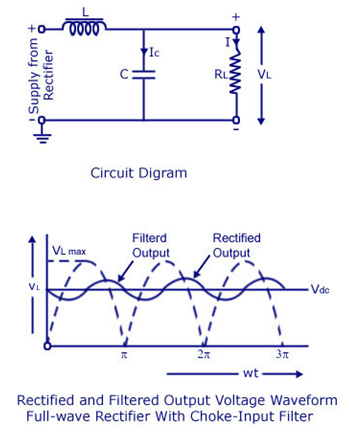Choke-input filter is explained below:

Choke-input filter consists of a choke L connected in series with the rectifier and a capacitor C connected across the load . This is also called the L-section filter because in this arrangement inductor and capacitor are connected, as an inverted L. In the figure, only one filter section is shown. But several identical sections are often employed to improve the smoothing action. (The choke L on the input side of the filter readily allows dc to pass but opposes the flow of ac components because its dc resistance is negligibly small but ac impedance is large. Any fluctuation that remains in the current even after passing through the choke are largely by-passed around the load by the shunt capacitor because Xc is much smaller than RL. Ripples can be reduced effectively by making XL greater than Xc at ripple frequency. However, a small ripple still remains in the filtered output and this is considered negligible if it is less than l%. The rectified and filtered output voltage waveforms from a full-wave rectifier with choke-input filter are shown in the figure above.

4 pi-SECTION FILTER: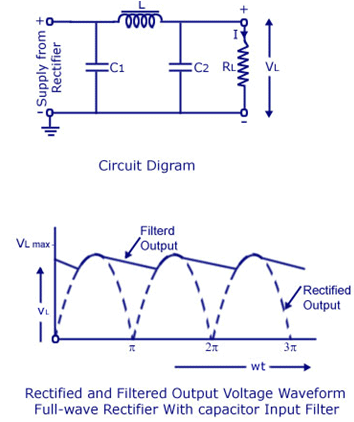Capacitor-Input or Pi-Filter.

Such a filter consists of a shunt capacitor C1 at the input followed by an L-section filter formed by series inductor L and shunt capacitor C2. This is also called the n-filter because the shape of the circuit diagram for this filter appears like Greek letter n (pi). Since the rectifier feeds directly into the capaci­tor so it is also called capacitor input filter.

As the rectified output is fed directly into a ca­pacitor C1 such a filter can be used with a half-wave rectifier (series inductor and L-section filters cannot be used with half-wave rectifiers). Usually electrolytic capacitors are used even though their capacitances are large but they occupy minimum space. Usually both capacitors C1 and C2 are enclosed in one metal container. The metal container serves as the common ground for the two capacitors.

A capacitor-input or pi- filter is characterized by a high voltage output at low current drains. Such a filter is used if, for a given transformer, higher voltage than that can be obtained from a L-section filter is required and if low ripple than that can be obtained from a shunt capacitor filter or L-section filter is desired. In this filter, the input capacitor C1 is selected to offer very low reactance to the ripple frequency. Hence major part of filtering is accomplished by the input capacitor C1Most of the remaining ripple is removed by the L-section filter consist­ing of a choke L and capacitor C2.)

The action of this filter can best be understood by considering the action of L-section filter(formed by L and C2), on the triangular output voltage wave from the input capacitor C1. The charging and discharging action of input capacitor C1 has already been discussed. The output voltage is roughly the same as across input capacitor C1 (Vc1 - dc drop across  the inductor). The ripples contained in this output are reduced further by L-section filter. The output voltage of pi-filter falls off rapidly with the increase in load-current and, therefore, the voltage regulation of this filter is very poor.

SALIENT FEATURES OF L-SECTION AND pi-FILTERS.

1. In pi-filter, the dc output voltage is much larger than that can be obtained from an L-section filter with the same input voltage.

2. In pi-filter, ripples are less in comparison to those in shunt capacitor or L-section filter. So choke of smaller inductance value is required in a pi-filter in comparison to that required in L-section filter.

3. In pi-filter, the capacitor is to be charged to the peak value hence the R.M.S current in supply transformer is larger as compared in case of L-section filter.

4. Voltage regulation in case of pi-filter is very poor, as already mentioned. So n-filters are suitable for fixed loads whereas L-section filters can work satisfactorily with varying loads provided a minimum current is maintained.

5. In case of a pi-filter PIV is larger than that in case of a L-section filter.

COMPARISON OF FILTERS

1) A capacitor filter provides Vm volts at less load current. But regulation is poor.

2) An inductor filter gives high ripple voltage for low load currents. It is used for high load currents.

3) L – Section filter gives a ripple factor independent of load current. Voltage Regulation can be improved by use of bleeder resistance.

4) Multiple L – Section filter or π filters give much less ripple than a single L–Section filter.

The document Filters Notes | Study Analog Electronics - Electrical Engineering (EE) is a part of the Electrical Engineering (EE) Course Analog Electronics.
All you need of Electrical Engineering (EE) at this link: Electrical Engineering (EE)Use Code STAYHOME200 and get INR 200 additional OFF

## Analog Electronics

11 videos|57 docs|97 tests

Track your progress, build streaks, highlight & save important lessons and more!

,

,

,

,

,

,

,

,

,

,

,

,

,

,

,

,

,

,

,

,

,

;